# Solve for Y(s), the Laplace transform of the solution y(t) to the initial value problem below....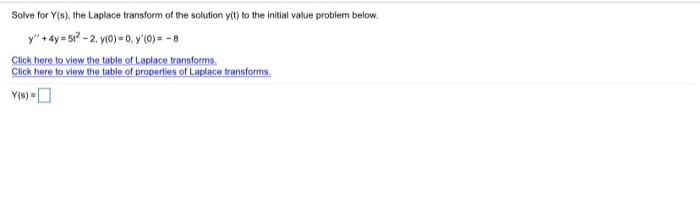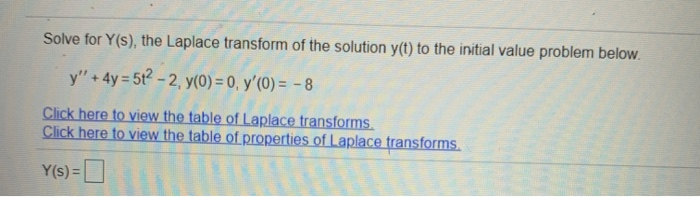Solve for Y(s), the Laplace transform of the solution y(t) to the initial value problem below. y" + 4y = 512 - 2. y(0)=0, 7(0) = -8 Click here to view the table of Laplace transforms Click here to view the table of properties of Laplace transforms.
Solve for Y(s), the Laplace transform of the solution y(t) to the initial value problem below. y" + 4y = 5t2 - 2. y(0) = 0, y'(O) = - 8 Click here to view the table of Laplace transforms. Click here to view the table of properties of Laplace transforms. Y(s) =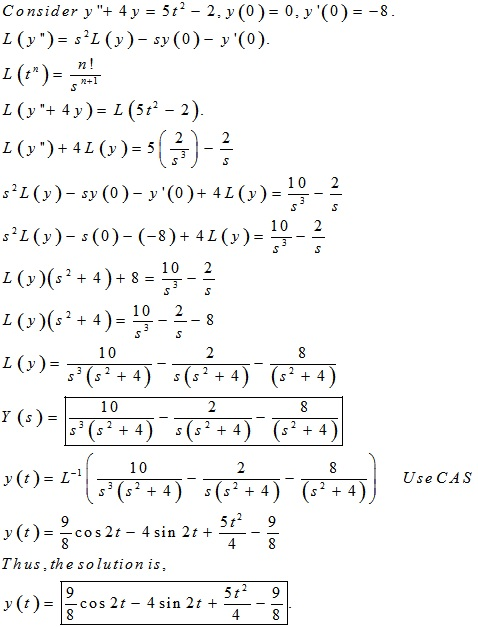##### Add Answer of: Solve for Y(s), the Laplace transform of the solution y(t) to the initial value problem below....
Similar Homework Help Questions
• ### Solve for Y(s), the Laplace transform of the solution yct) to the initial value problem below....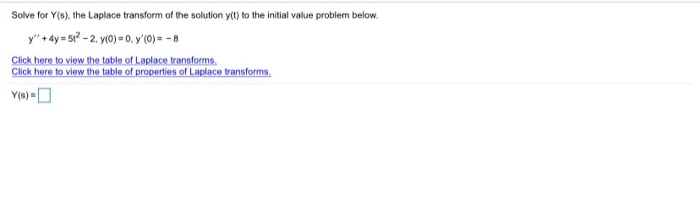Solve for Y(s), the Laplace transform of the solution yct) to the initial value problem below. y" + 4y = 512 - 2. y(0)=0, 7(0) = -8 Click here to view the table of Laplace transforms Click here to view the table of properties of Laplace transforms.

• ### Solve for Y(s), the Laplace transform of the solution y(t) to the initial value problem below...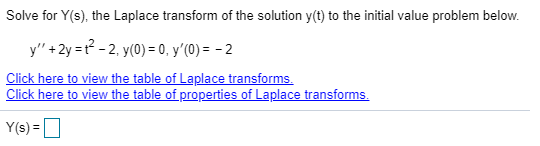Solve for Y(s), the Laplace transform of the solution y(t) to the initial value problem below y+2yt22, y(0) = 0, y'(0) = - 2 Click here to view the table of Laplace transforms Click here to view the table of properties of Laplace transforms Y(s)= Solve for Y(s), the Laplace transform of the solution y(t) to the initial value problem below y+2yt22, y(0) = 0, y'(0) = - 2 Click here to view the table of Laplace transforms Click here...

• ### Solve for y(s), the Laplace transform of the solution y(t) to the initial value problem below....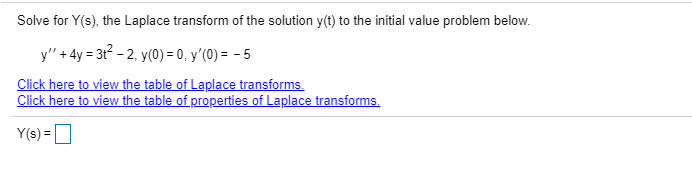Solve for y(s), the Laplace transform of the solution y(t) to the initial value problem below. y" + 4y = 32 - 2, y(0) = 0, y'(0) = -5 Click here to view the table of Laplace transforms. Click here to view the table of properties of Laplace transforms. Y(s)-

• ### Solve for y(s), the Laplace transform of the solution y(t) to the initial value problem below....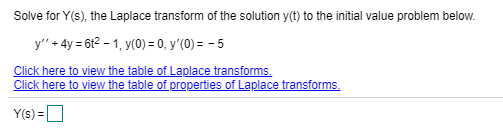Solve for y(s), the Laplace transform of the solution y(t) to the initial value problem below. y'' + 4y = 612 - 1, y(0) = 0, y'(0) = -5 Click here to view the table of Laplace transforms. Click here to view the table of properties of Laplace transforms. Y()=

• ### Solve for Y(s), the Laplace transform of the solution y(t) to the initial value problem below....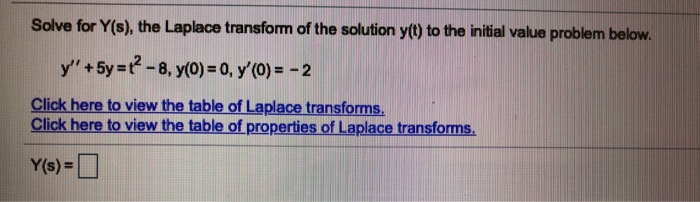Solve for Y(s), the Laplace transform of the solution y(t) to the initial value problem below. y' +5y =12 - 8, 7(0) = 0, y'(0) = -2 Click here to view the table of Laplace transforms. Click here to view the table of properties of Laplace transforms. Y(s)=0

• ### Solve for Y(s), the Laplace transform of the solution y(t) to the initial value problem below....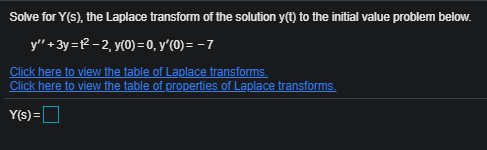Solve for Y(s), the Laplace transform of the solution y(t) to the initial value problem below. y" + 3y = {2-2, y(0) = 0, y'(0) = -7 Click here to view the table of Laplace transforms. Click here to view the table of properties of Laplace transforms. Y(s)=

• ### Solve for Y(s), the Laplace transform of the solution y(t) to the initial value problem below....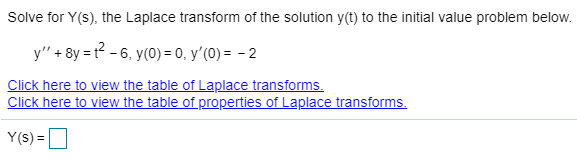Solve for Y(s), the Laplace transform of the solution y(t) to the initial value problem below. y" + 8y = 2 - 6, y(0) = 0, y' (O) = -2 Click here to view the table of Laplace transforms. Click here to view the table of properties of Laplace transforms. Y(S) =

• ### Solve for Y(s), the Laplace transform of the solution y(t) to the initial value problem below....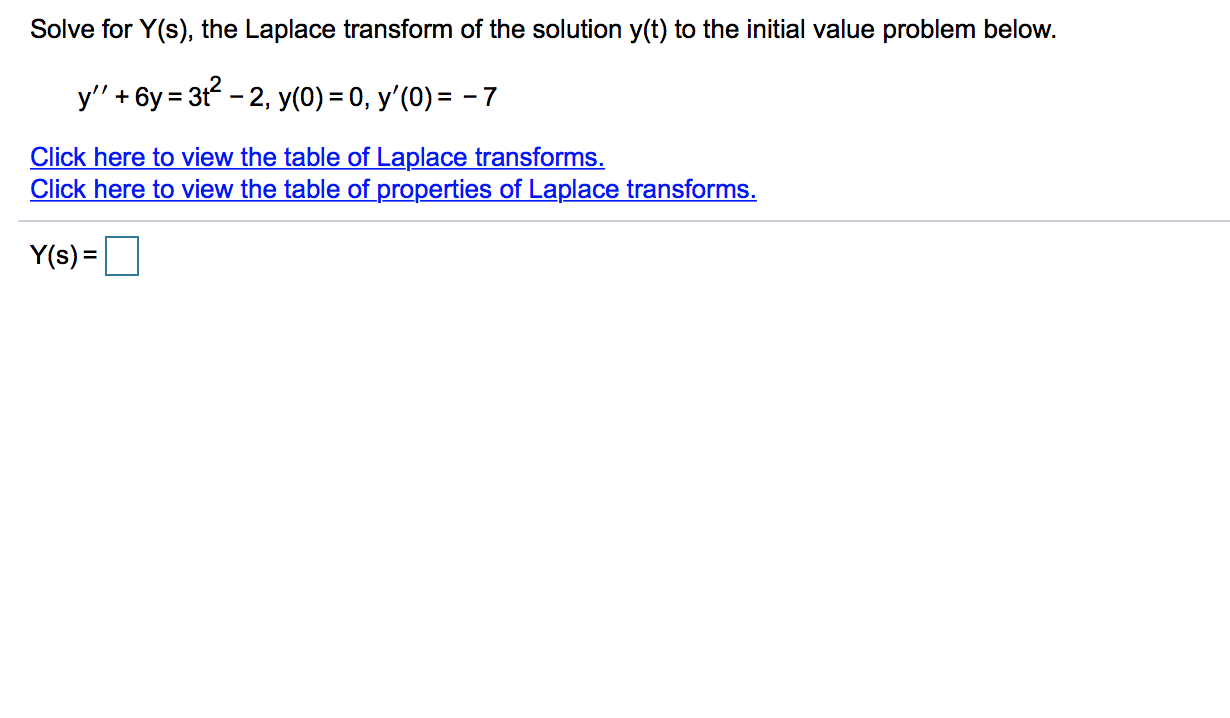Solve for Y(s), the Laplace transform of the solution y(t) to the initial value problem below. y' + 6y = 312 - 2, y(0) = 0, y'(0) = - 7 Click here to view the table of Laplace transforms. Click here to view the table of properties of Laplace transforms. Y(s)-

• ### Solve for Y(s), the Laplace transform of the solution y(t) to the initial value problem below....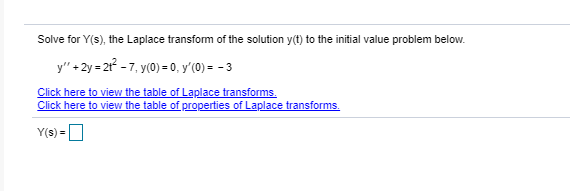Solve for Y(s), the Laplace transform of the solution y(t) to the initial value problem below. y" +2y = 2+2 -7,y(0) = 0, y'(0) = - 3 Click here to view the table of Laplace transforms. Click here to view the table of properties of Laplace transforms. Y(s)-

• ### Solve for Y(s), the Laplace transform of the solution y(t) to the initial value problem below....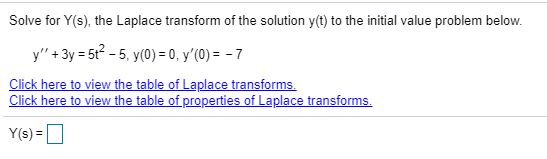Solve for Y(s), the Laplace transform of the solution y(t) to the initial value problem below. y" + 3y = 52 - 5, y(0) = 0, y'(0) = -7 Click here to view the table of Laplace transforms. Click here to view the table of properties of Laplace transforms. Y(s)=

Free Homework App